## Online Education for RS Aggarwal Class 9 Solutions Chapter 1 Real Numbers Ex 1A

These Solutions are part of RS Aggarwal Solutions Class 9. Here we have given RS Aggarwal Solutions Class 9 Chapter 1 Real Numbers Ex 1A.

Other Exercises

Question 1.
Solution:
A number in the form of $$\frac { p }{ q }$$ where p and q are integers and q ≠ 0, is called a rational numberare all rational numbers.

Question 2.
Solution:
The given rational number are represented on a number line on given below :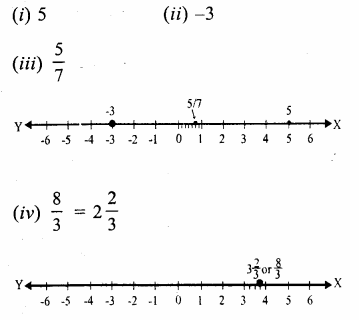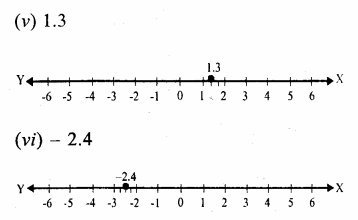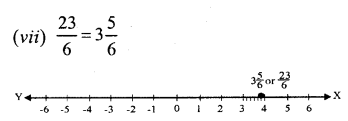Question 3.
Solution:
We know that, if a and b are two rational numbers, then a rational number between a and b will be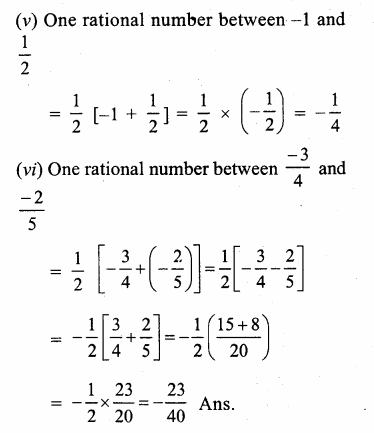Question 4.
Solution:
Here, n = 3, x = $$\frac { 1 }{ 5 }$$, y = $$\frac { 1 }{ 4 }$$Question 5.
Solution:
Here, n=5, x = $$\frac { 2 }{ 5 }$$, y = $$\frac { 3 }{ 4 }$$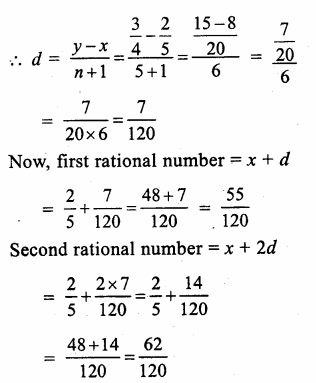Question 6.
Solution:
Here, n = 6, x = 3, y = 4Question 7.
Solution:
Here, n = 16, x = 2.1, y = 2.2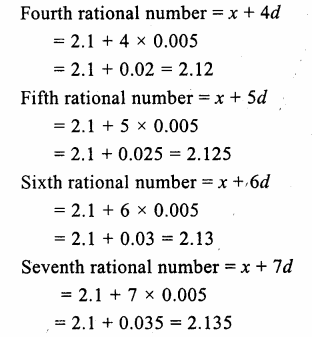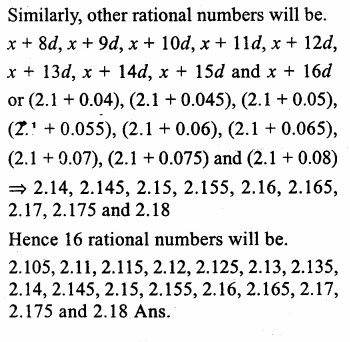Hope given RS Aggarwal Solutions Class 9 Chapter 1 Real Numbers Ex 1A are helpful to complete your math homework.

If you have any doubts, please comment below. Learn Insta try to provide online math tutoring for you.